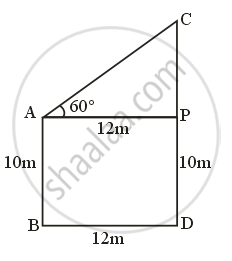Share

# Two Buildings Are Facing Each Other on a Road of Width 12 Metre. from the Top of the First Building, Which is 10 Metre High, the Angle of Elevation of the Top of the Second is Found to Be 60°. - Geometry

ConceptHeights and Distances

#### Question

Two buildings are facing each other on a road of width 12 metre. From the top of the first building, which is 10 metre high, the angle of elevation of the top of the second is found to be 60°. What is the height of the second building ?

#### SolutionLet AB and CD be two building, with

AB = 10 m

And angle of elevation from top of AB to top of CD = ∠CAP = 60°

Width of road = BD = 12 m

Clearly, ABDP is a rectangle

With

AB = PD = 10 m

BD = AP = 12 m

And APC is a right-angled triangle, In ∆APC

tan theta = "Perpendicular"/"Base" = "CP"/"AP"

=> tan 60°  = "CP"/12

=> sqrt3 = "CP"/12

⇒ CP = 12√3 m

Also,

CD = CP + PD = (123 + 10) m

Hence, height of other building is (10 + 123 m).

Is there an error in this question or solution?

#### APPEARS IN

Balbharati Solution for Balbharati Class 10 Mathematics 2 Geometry (2018 to Current)
Chapter 6: Trigonometry
Practice set 6.2 | Q: 3 | Page no. 137

#### Video TutorialsVIEW ALL 

Solution Two Buildings Are Facing Each Other on a Road of Width 12 Metre. from the Top of the First Building, Which is 10 Metre High, the Angle of Elevation of the Top of the Second is Found to Be 60°. Concept: Heights and Distances.
S